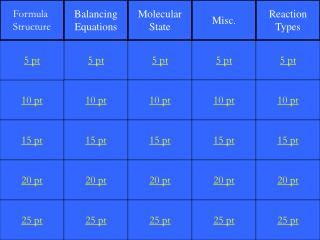DownloadDownload Presentation10 pt

# 10 pt

Télécharger la présentation## 10 pt

- - - - - - - - - - - - - - - - - - - - - - - - - - - E N D - - - - - - - - - - - - - - - - - - - - - - - - - - -
##### Presentation Transcript

1. Formula Structure Balancing Equations Molecular State Misc. Reaction Types 5 pt 5 pt 5 pt 5 pt 5 pt 10 pt 10 pt 10 pt 10 pt 10 pt 15 pt 15 pt 15 pt 15 pt 15 pt 20 pt 20 pt 20 pt 20 pt 20 pt 25 pt 25 pt 25 pt 25 pt 25 pt

2. The result of a chemical reaction is called a

3. Product

4. Coefficient

5. When an arrow () is used in a reaction, on which side are the reactants found?

6. Left

7. The “small” numbers that describe the amount of one element is called a

8. Subscript

9. False

10. Fe + O2 Fe2O3Balance the equation.

11. 4Fe + 3O2 2Fe2O3

12. HgO Hg + O2Balance the equation.

13. 2HgO  2Hg + O2

14. How many molecules of Cl2 will react with 2 atoms of Na?

15. 1Daily Double!!! In 2NH3, 3 is called the…

16. 3

17. What is the symbol for “solid”?

18. s

19. What is the symbol for “gas”?

20. g

21. What is the symbol for a liquid?

22. l

23. What is the most common state of carbon dioxide?

24. gas

25. What is the symbol for an aqueous solution?

26. aq

27. 4 H2SO4How many hydrogen atoms are there?

28. 8

29. True

30. What is the “matchmaker” that increases the rate of a chemical reaction without being chemically changed itself?

31. Catalyst

32. True

33. What is the chemical formula for iron (III) oxide?

34. Fe2O3

35. 2 AlCl3 2 Al + 3 Cl2 What type of reaction is represented by the equation?

36. Decomposition

37. AgCl + Na(NO3)

38. HBr + NaOH H2O + NaBrWhat type of reaction is represented?

39. Double Displacement

40. 2 Na + Cl2 NaClWhat type of reaction is represented?

41. Synthesis

42. Zn + 2 HCl ZnCl2 + H2What type of reaction is represented?

43. Single Displacement

44. H2O2 O2 + H2What type of reaction is represented?